https://riskmatrix.co/blogs/risk-matrix-knowledge-bank.atom Riskfiniti - Risk Matrix Knowledge Bank 2019-02-12T14:52:00-06:00 Riskfiniti https://riskmatrix.co/blogs/risk-matrix-knowledge-bank/how-not-to-design-a-risk-matrix-government-of-western-australia 2019-02-12T14:52:00-06:00 2019-02-17T20:48:27-06:00 How Not to Design a Risk Matrix – Government of Western Australia’s Risk Matrix Peter Carr Today, I stumbled across one of the most badly designed risk matrices I have ever encountered. It has been published by the Government of Western Australia, Small Business Development Corporation, and is offered as a guiding example to small businesses (Ref. 1, 2):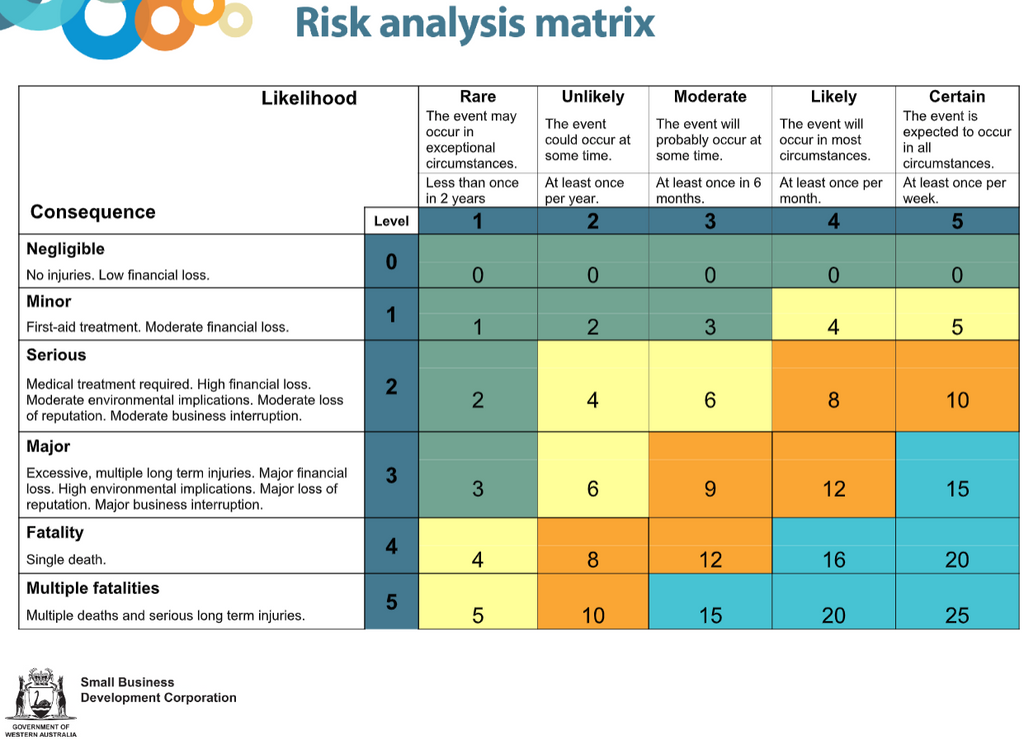The risk matrix contains several major flaws.

## Scales with Inappropriate Ranges

The Consequence scale ranges as high as “Multiple Fatalities” but the Likelihood scale only goes as low as “Less than once in 2 years.” The user needs to be able to determine from the risk matrix when the likelihood of a multiple-fatality event has been reduced to a broadly acceptable level. One criterion for determining the limit for multiple-fatality events is F = 10-3/N2 per year where N is the number of fatalities (this criterion has been applied to a number of activities in the Netherlands such as the national airport, Shiphol). At this point, you may see another problem with the scales – vagueness – just how many fatalities are envisaged in the multiple-fatality consequence category? Suppose this category corresponds to 2 to 10 fatalities. Then, on the Dutch criterion, the frequency limit would be 2.5 x 10-4 (once in 4,000 years) to 1.0 x 10-4 per year (once in 10,000 years). Thus, the likelihood scale should go down very much lower than once in 2 years in order to accommodate multiple-fatality scenarios.

## Scales with Strange Gaps

Suppose an event is predicted to occur once in two years on average. This is a higher frequency than for category 1 (defined as less than once in two years) but lower frequency than for category 2 (defined as at least once per year). Thus, there is no category in which to place the event that is expected to occur, on average, once in two years.

## Likelihood Categories that are Almost Certain

From the Likelihood categories definitions, events in likelihood categories 2 to 5 occur quite frequently – from once per year (category 2) to every week (category 5). These events are almost certain to occur. Events that are almost certain to occur – or have already occurred – are better treated as issues rather than risks. The term "risk" should be reserved for unwanted events that are possible but not those that may be confidently anticipated to arise. This avoids cluttering the risk register with events that occur routinely.

## Zero Width Categories

Consequence category 4 applies to the event of exactly 1 fatality so has zero width. It would be better to combine it with category 5 and call it, say, “1 to 10 fatalities.” This avoids giving the risk assessment team the challenge of distinguishing scenarios that cause a single fatality from those that cause two or more fatalities.

## Inappropriate Risk Criteria

Consider a multiple-fatality event that is predicted to occur once in 3 years, on average. The risk matrix classifies this as a “moderate” risk that “may require corrective action.” Of course, such a severe event with such a high frequency should be placed in the highest category of risk and described as completely unacceptable.

## Category Labels Treated as Quantitative Measures

The category labels 0 to 5 do not express the magnitude of the likelihood or the consequence. They could equally have been labeled A to F. Just because the labels look like numbers does not mean that they can be treated as numbers. The author of this risk matrix has multiplied these numerals, which are really just labels, in a pretense at producing a measure of risk. Then, for example, “Extreme” risk is defined as a product from 15 to 25. This oversimplified approach to designing a risk matrix will nearly always result in a risk matrix with an inappropriate coloring pattern, i.e. it will misclassify risks. This is a grievous type of error in risk matrix construction to which I have previously devoted an entire article.

I find it shocking that such a poorly designed risk matrix should have been presented as official guidance to small businesses by the Government of Western Australia.

## External References

]]>
https://riskmatrix.co/blogs/risk-matrix-knowledge-bank/how-not-to-design-a-risk-matrix-cox-s-axioms 2018-05-28T17:00:00-05:00 2018-05-28T18:16:33-05:00 How Not to Design a Risk Matrix—Cox’s Axioms Peter Carr More

]]>
Introduction

The most widely cited paper on risk matrix design is by Cox (2008). It includes a design methodology based on three axiomatic constraints that Cox suggests risk matrices should satisfy. Cox applies the axioms to produce designs for risk matrices of size 3x3, 4x4, and 5x5. In this article, we review the method and show that it leads to results that are not useful in practice.

## Cox's Axioms

In explaining the design method of Cox, we will adopt “probability” and “impact” as the default names of the two axes of the risk matrix.

Cox assumes that the two axes range from 0 to 1 and are equally subdivided into a number of intervals. For example, for a 4x4 matrix, the axes would be as follows: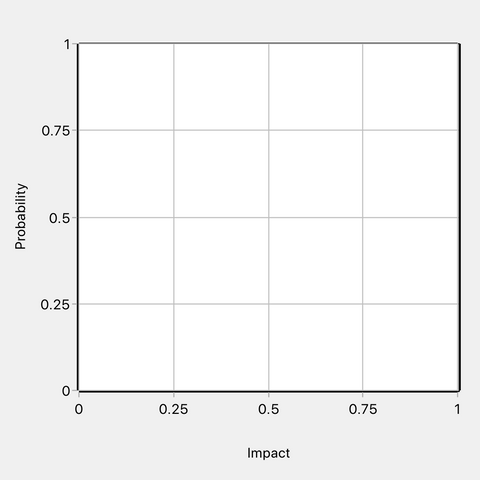Figure 1: 4x4 risk matrix axes

On the probability axis, 0 represents impossibly while 1 represents certainty. On the consequence axis, 0 represents no impact and 1 represents the worst possible adverse impact. The consequence axis tick mark values could be multiplied by any positive scaling factor without changing the mathematical structure.

Cox assumes that risk is calculated as a function of probability and consequence, for example, as the product of the two variables.

Cox proposes that a risk matrix coloring pattern must satisfy three axiomatic constraints. The three constraints he calls Weak Consistency, Betweenness and Consistent Coloring. The following definitions are from Cox’s paper (note he assumes a risk matrix with three colors—red for the top risk category, green for the bottom category, and yellow as an intermediate color):

DEFINITION OF WEAK CONSISTENCY: A risk matrix with more than one “color” (level of risk priority) for its cells satisfies weak consistency with a quantitative risk interpretation if points in its top risk category represent higher quantitative risks than points in its bottom category.

DEFINITION OF BETWEENNESS: A risk matrix satisfies the axiom of betweenness if every positively sloped line segment that lies in a green cell at its lower (left) end and in a red cell at its upper (right) end passes through at least one intermediate cell (meaning one that is neither green nor red) between them.

DEFINITION OF CONSISTENT COLORING: (1) A cell is red if it contains points with quantitative risks at least as high as those in other red cells (and does not contain points with quantitative risk as small as those in any green cell). (2) A cell is colored green if it contains some points with risks at least as small as those in other green cells (and does not contain points with quantitative risks as high as those in any red cell). (3) A cell is colored an intermediate color (neither red nor green) only if either (a) it lies between a red cell and a green cell; or (b) it contains points with quantitative risks higher than those in some red cells and also points with quantitative risks lower than those in some green cells.

According to Cox, the three axioms taken together imply that there is only one possible coloring for a 3x3 or 4x4 matrix, and only two possible colorings for a 5x5 matrix. Let’s take a 4x4 risk matrix as an example. Cox states that the only possible coloring is as shown in Figure 2 below.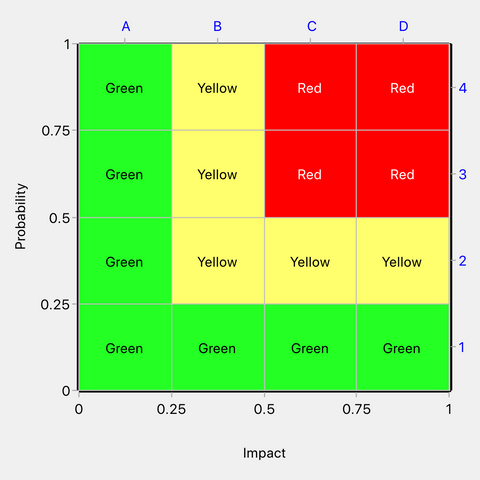Figure 2: The only permissible coloring of a 4x4 risk matrix according to Cox

A good way to understand the three axioms is to consider how the above risk matrix satisfies them.

Weak Consistency. Points in the green cells correspond to quantitative risks from 0 to 0.25. Risks in the red cells correspond to quantitative risks from 0.25 to 1. Points in the top risk category therefore represent risks that are higher than (or equal to) those in the bottom category. Cox’s definition of Weak Consistency (see above) does not mention the possibility that risks in the bottom and top categories could be equal but, from his 4x4 example, it appears that this is acceptable and does not violate Weak Consistency.

Betweenness. It is not possible to draw a line from a green cell to a red cell without passing through at least one yellow cell. Therefore, Betweenness is satisfied.

Consistent Coloring. Inspection shows that the Consistent Coloring axiom is satisfied. But note, the Consistent Coloring axiom contains within it several rules numbered (1), (2), (3)(a) and (3)(b). An interesting feature is that according to Consistent Coloring rule (2), cell B2 should have been green but it has been taken as yellow to satisfy Consistent Coloring rule (3)(a). Rule (3)(a) appears to have been included to allow Betweenness to take precedence over what would otherwise be deemed consistent coloring. In other words, it appears that rules (2) and (3)(a) are in conflict for cell B2, with rule (3)(a) being used as the final determinant of its color.

Note that the Consistent Coloring axiom is very restrictive over when a cell may be colored yellow. It may be yellow according to Rule (3)(a) when it lies between a green cell and a red cell (i.e. when a yellow cell is introduced to satisfy Betweenness). A cell may also be yellow according to Rule (3)(b) when it contains points with quantitative risks higher than those in some red cells and also points with quantitative risks lower than those in some green cells. Due to the restrictive circumstances in which a cell may be colored yellow, many coloring patterns are impermissible, for example, that shown in Figure 3 below.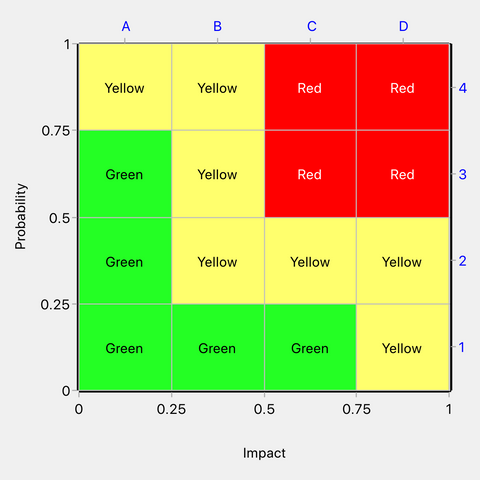Figure 3: An example of a coloring pattern that would be impermissible under the Cox axioms

The above matrix is impermissible under the Cox axioms because yellow cells A4 and D1 comply neither with Consistent Coloring rule (3)(a) nor with (3)(b).

Now we will ask what the one and only permissible design for a 4x4 matrix under the Cox axioms implies about risk priority levels. We have seen that green cells span risks from 0 to 0.25 and red cells span the range 0.25 to 1. Thus, when a risk falls in the red zone, we know it is larger than or equal to 0.25, and when it falls in the green zone it is less than or equal to 0.25. The matrix is therefore useful for discriminating between risks according to whether they are above or below the value of 0.25. We can see that the yellow zone overlaps with both green and red by spanning the risk range from 0.0625 to 0.5. When a risk falls in the yellow zone, we learn nothing about its relationship to a risk threshold of 0.25.

Cox does not explain how the yellow zone should be interpreted. Some points in the yellow cells correspond to risks as high as some points in red cells, while other points in yellow cells correspond to risks as low as some points in green cells. Yellow could be assumed to be an intermediate risk level between red and green but we can see that it overlaps with both red and green in terms of risk range, so it is not actually an intermediate level.

The Cox 4x4 risk matrix design is more or less compatible with the quantitative model shown in Figure 4 that classifies risks as red or green according to their relationship to an iso-risk contour of value 0.25. Simply color the cells that contain both red and green coloring as yellow and you will have the Cox design—except that Cox forces cell B2 to be yellow rather than green on account of Betweenness and the Consistent Coloring Rule (3)(a). Without forcing the color of cell B2, we would have the matrix in Figure 5, which is the same as Cox’s design except for cell B2.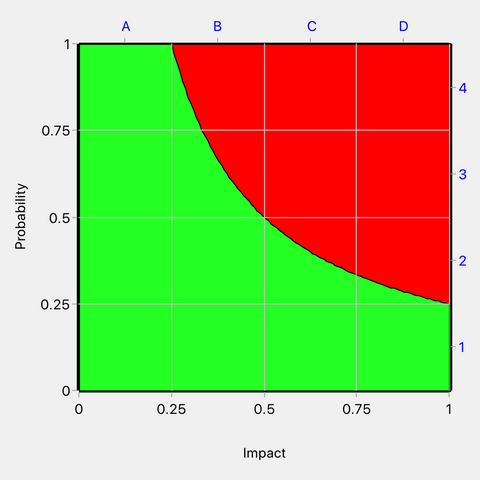Figure 4: A quantitative model compatible with the Cox 4x4 risk matrix design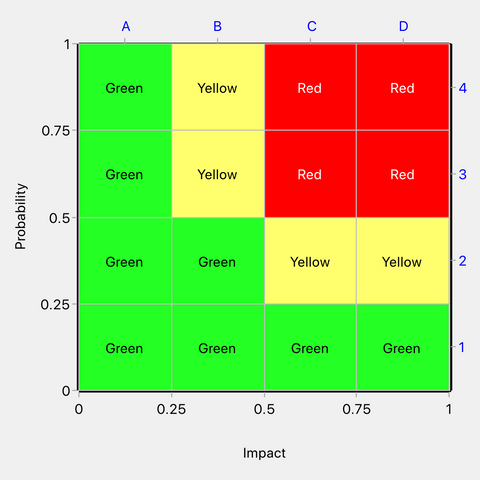Figure 5: Matrix compatible with the quantitative model in Figure 4

It seems that the only valid use of the Cox 4x4 matrix is to tell us whether a risk is above or below a threshold of 0.25. It will do this accurately when the risk falls in the red or green zone but provides no useful information when the risk falls in the yellow zone. Therefore, a logical development of the design is to approximate the yellow cells as either green or red. Let us approximate the yellow cells as red. Our design now evolves to: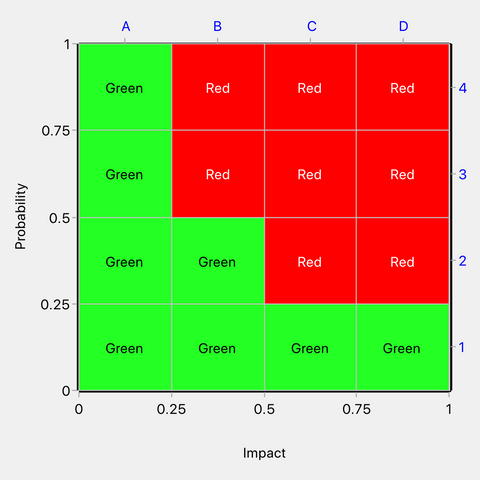Figure 6: Yellow cells approximated as red

Suppose that risk points are uniformly distributed in Figure 6. We can simulate a large number of such points and determine how many are correctly ranked according to the underlying model in Figure 4. We find that 90% of points will be correctly ranked and 10% will have their rank overestimated, which is a respectable performance for an approximate tool.

But the single risk level of 0.25 implied in the Cox 4x4 matrix is unlikely to be of any use to the decisionmaker. Risks in the domain of the matrix can range from 0 at the bottom left to 1 at top right. The decisionmaker might be interested in a value for the risk threshold anywhere in this range. Also, in practice, the decisionmaker will likely want to use more than one risk threshold, e.g. to divide risks into three or more categories. Cox’s 4x4 matrix design relates to a single risk threshold fixed at 0.25 by the application of the three axiomatic constraints.

## Conclusions

The three Cox axioms overly constrain risk matrix design resulting in a single implied risk threshold between the top and bottom risk categories. We demonstrated this to be the case for the 4x4 matrix, but it is also the case for the 3x3 and 5x5 risk matrix designs in the Cox paper.

The Cox axioms cannot be used to develop a design that is compatible with arbitrary risk threshold values specified by the decisionmaker.

The Cox axioms are not useful for practical risk matrix design.

## References

Cox, L. A., Jr. (2008). What's wrong with risk matrices? Risk Analysis, 28(2), 497-512. doi:10.1111/j.1539-6924.2008.01030.x

]]>
https://riskmatrix.co/blogs/risk-matrix-knowledge-bank/when-can-the-same-risk-matrix-be-used-with-different-axis-scales 2018-03-27T23:56:00-05:00 2018-05-26T19:22:30-05:00 When Can the Same Risk Matrix be Used with Different Axis Scales? Peter Carr More

]]>

The visual elements of any well-designed risk matrix are:

• A ratio scale for its probability (or frequency) axis
• A ratio scale for its consequence axis
• Contours of equal risk (iso-risk contours), which define the thresholds between the different risk priority levels
• A set of colors to visually differentiate the different risk priority levels

In addition, an algorithm is needed for deciding the color of cells that are intersected by one or more iso-risk contours. These cells, which we call “split cells” for brevity, are not located in a single risk priority level. Different parts of a split cell lie in different risk priority levels. Each split cell must be allocated to just one of the risk priority levels that it straddles. Quick Risk Matrix offers a choice of several algorithms by which to determine the coloring of the split cells.

Now, many organizations like to use a single risk matrix coloring pattern but in conjunction with different axis scales. Let’s invent an example.

Suppose an organization develops a risk matrix for the consequence type of environmental damage as shown in Figure 1 and wishes to apply the same matrix coloring scheme to the consequence type of financial loss. What are the circumstances in which it may do so?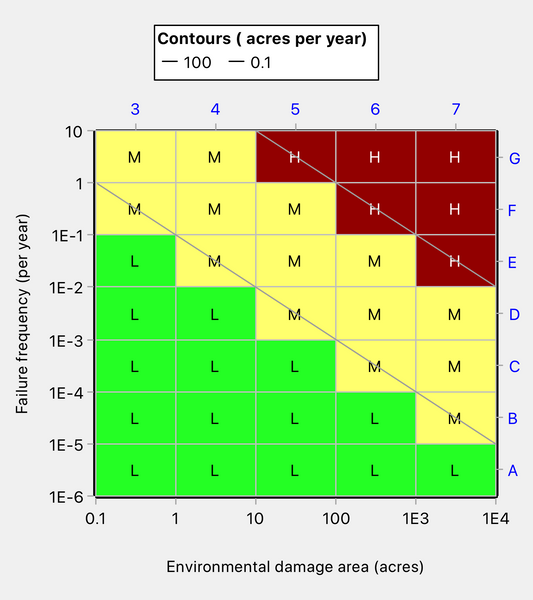Figure 1: Risk matrix for environmental damage

In switching the horizontal axis from environmental damage to financial loss, there will be no impact on the matrix coloring scheme if the tick mark values on the horizontal axis are multiplied by a factor and if the iso-risk contours, which define the risk priority levels, are multiplied by the same factor. Let’s say that the factor will be 100. Then the risk matrix for financial loss is as shown in Figure 2, and it has the same coloring pattern as the one for environmental damage shown in Figure 1.Figure 2: Risk matrix for financial loss

So far, so good. Our fictitious organization can have a standard risk matrix coloring as shown in Figure 1 or 2 and use it in relation to both environmental damage and financial loss.

But what if the organization feels that an expected annual financial loss of \$10 is trivially low? Perhaps it would be willing to categorize risks as Low if the annual expected loss is under \$100? The lowest iso-risk contour will then shift upwards and the risk matrix coloring will change as shown in Figure 3 below. There is no fundamental reason why, when the consequence scale changes by a multiplicative factor, that the iso-risk contour values should change by the same factor. For example, it might well be that the organization is relatively more tolerant of financial loss than it is of environmental damage.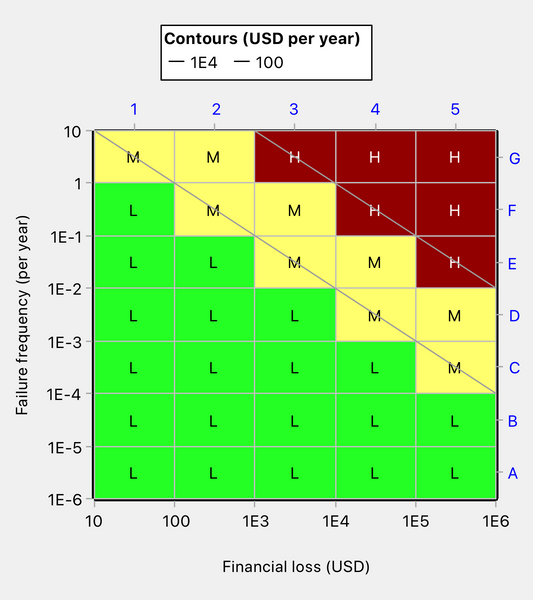Figure 3: Risk matrix coloring has changed as a result of a change in an iso-risk contour value

Suppose now that the organization feels that consequence category 2 would be more useful if it were defined as the interval [100, 500], rather than as [100, 1000]. The risk matrix coloring pattern now changes as shown in Figure 4. On account of the redefinition of the category, the three cells marked *** have changed in color.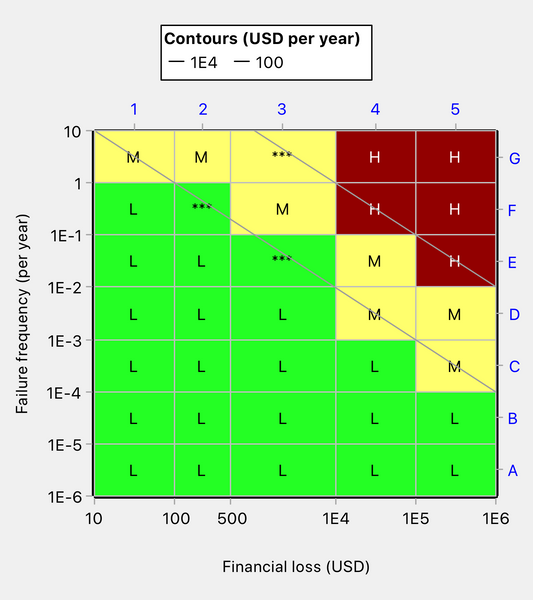Figure 4: Risk matrix coloring changed as a result of redefining consequence category 2

We have demonstrated that changes to tick mark values (other properties of the matrix remaining constant) may change the coloring pattern. Tick mark value changes do not necessarily change the coloring pattern, but they will often do so.

We have also demonstrated that changing the risk values that define the risk priority levels will change the coloring.

If we use a different algorithm for determining the color of split cells, the risk matrix coloring pattern may again change. For example, we might want to use the “Round Up” algorithm for environmental damage to ensure that all errors of risk mapping are on the safe side, but the “Predominant Color” algorithm for financial loss since we may be less concerned about underestimating risk when it is merely a matter of money rather than environmental harm. Why don’t we always use the round Up method? Because it is usually less accurate than the Predominant Color method. Please see other posts in this blog for a full understanding of the different coloring algorithms for split cells.

## Conclusions

We have shown that the same risk matrix coloring pattern can be applied with different axis scales only under very limited circumstances.

In our experience, many organizations have a standard risk matrix that they use in conjunction with diverse axis scales.  Unless the matrix and the scales have been very carefully designed, the matrix may be invalid in relation to some or even all of the scales.

If a standard risk matrix is wanted, the safest approach is to use Quick Risk Matrix to produce a risk matrix design for each set of axis scales and to verify that the design is, in fact, the same in each case.

It is also a reasonable and probably more flexible approach to have several risk matrices in the organization, each tailored to different types of consequence.

]]>
https://riskmatrix.co/blogs/risk-matrix-knowledge-bank/benchmarking-risk-matrix-performance 2018-03-27T23:44:00-05:00 2018-05-26T19:21:29-05:00 Benchmarking Risk Matrix Performance Peter Carr More

]]>
In this post, we discuss the question of how to benchmark the performance of a risk matrix, so that different risk matrix designs may be compared. In so doing, we will develop some tips for the more accurate design of risk matrices.

This post assumes that the reader has read our earlier blog posts and is familiar with the design methodology used in Quick Risk Matrix.

After starting this article, we found ourselves unavoidably discussing two methods of risk matrix design, in addition to the method used in Quick Risk Matrix. We will demonstrate that the former methods  (i.e.. the non Quick Risk Matrix methods)  are not defensible.

We shall create our examples using Quick Risk Matrix Premium, which includes a performance benchmarking tool.

## Literature Review

There is very little in the literature on the subject of benchmarking risk matrix performance. As far as we are aware, the only papers of note are those by Cox (Ref. 1), Xing Hong (Ref. 2), and Li, Bao and Wu (Ref. 3).

Cox investigated risk matrices up to size 5 x 5 with three colors and equally subdivided axis scales ranging from 0 to 1. He concluded that risk matrices typically have poor resolution. He states "Typical risk matrices can correctly and unambiguously compare only a small fraction (e.g., less than 10%) of randomly selected pairs of hazards." That is a startling conclusion. However, his assumptions are pessimistic. For example, he assumes that when two risks fall in the same risk priority level (i.e. they are located in cells of the same color), there is only a 50% probability of ranking them in the correct order. His assumption is correct when both risk points are located in the same cell but not correct when the two points lie in different cells (unless the decisionmaker is using blind guesswork!). We shall recommend a method in this post for breaking ties when two risks have equal priority levels. In addition, Cox generates his figure of a 90% error rate by using a specific risk matrix and with the assumption that the plotted risks lie in the worst possible positions in the risk matrix.

Cox also proposes a design methodology for matrices based on axioms that he calls weak consistency, betweenness and color consistency. He illustrates the methodology for matrices with three colors ranging from size 3 x 3 to 5 x 5. He develops the surprising conclusion that there is only one possible coloring pattern for a 3 x 3 or 4 x 4 matrix and only two possible colorings for a 5 x 5 matrix. We won't go into detail here but we will point out that the limited number of colorings stems from axioms that are overly restrictive. The rational risk matrix designer will want to specify the risk values that define the thresholds between the different risk priority levels. There is nowhere in the Cox methodology for input of the risk thresholds. Instead, the coloring that flows from the axioms implies the thresholds, which is putting the cart before the horse!

Li et al. propose a design methodology that relaxes Cox's axioms. They call their methodology the Sequential Updating Approach (SUA). They impose a condition that for a cell A to have a higher risk priority level than a cell B, the probability that a random point in A has higher quantitative risk than a random point in B must be greater than a certain value, alpha (0.5 <=  alpha <= 1). An assumption is required for the color of the bottom left cell. Then, by an iterative process, the colors of all the other cells may be determined. The authors show that for a given value of alpha, and given subdivisions for the axes, there is a unique risk matrix coloring. The approach maximizes the number of colors (risk priority levels) in the matrix for a given value of alpha. The higher the value of alpha, the fewer the number of colors that will appear in the matrix. The SUA methodology, like the Cox methodology, pays no regard to the risk threshold values that the risk matrix designer might want to use. The Sequential Updating Approach and the Cox methodology are both indefensible for this reason.

Li et al. use two measures to assess the performance of risk matrices, which they call Probability of Elimination Error (PEE) and Probability of Wrong Pairs (PWP).  They calculate these two measures by generating random pairs, assuming that probability and consequence are uniformly distributed and independent. If the two points within a pair lie in different risk priority levels, and the ranking based on quantitative risk differs from the ranking based on risk priority level, they count that as both a PWP error and a PEE. If the two points lie in the same risk priority level, then the two points will be ranked equally and some procedure (applied by the end user of the risk matrix) must be assumed if the tie is to be broken. It is not clear what tie-breaking procedure was assumed. A PEE is counted when the tie-breaking procedure fails to give the correct risk ranking.

The authors describe PEE as a measure of resolution and PWP as a measure of accuracy. In worked examples, the authors found that as the value of alpha increased, the number of colors in the matrix decreased, the PEE increased and the PWP decreased.

Note. The term PEE appears to have been coined by Xing Hong (Ref. 2).

## Benchmarking Measures in Quick Risk Matrix

The performance benchmarking tool in Quick Risk Matrix Premium uses three measures of performance, in contrast to two used by Li et al. and one by Cox.

The measures used in Quick Risk Matrix are:

• Accuracy of Mapping
• Probability of Elimination Error (PEE)
• Probability of Rank Reversal (PRR)

We will explain each of these measures below.

### Accuracy of Mapping

As explained in more detail in other posts in this blog, Quick Risk Matrix treats a risk matrix as an approximation to a risk graph. The risk graph shows without error how probability and consequence map to risk priority level. The risk matrix will inevitably make mapping errors and one of the purposes of risk matrix design is to make these errors as few as possible, i.e. the risk matrix should be a good approximation to the risk graph.

The performance benchmarking tool in Quick Risk Matrix Premium assesses the mapping accuracy of a risk matrix by generating a large number of random risk points and counting how often the risk points are mapped correctly to risk priority level. The risk graph determines what constitutes correct mapping. In generating the random points, probability and consequence are assumed uniformly distributed when the matrix axes are linear and log-uniformly distributed when the matrix axes are logarithmic. The probability and consequence are taken to be correlated with a Spearman correlation coefficient input by the program user in the range -1 to +1. A Spearman coefficient of 0 corresponds to independence between the variables.

The results are presented as:

• Percentage correctly mapped
• Percentage mapped to a risk priority level that is too high
• Percentage mapped to a risk priority level that is too low

Accurate mapping is arguably the most important characteristic of a well-designed risk matrix.

### Probability of Elimination Error (PEE)

The concept of PEE arises in the scenario that a decisionmaker may have two risks but can only afford to eliminate one of them. Which one should be eliminated? While factors unrelated to the risk matrix will go into such a decision, it would certainly be helpful to be able to rank risks on the basis of the risk matrix, even for risks located in the same risk priority level.

Our definition of PEE is the same as employed by Xing Hong and Li et al. However, it is important to state what tie-breaking procedure is assumed when the two points in a pair lie in the same risk priority level. So we will now discuss some possible tie-breaking methods.

#### Possible Tie-Breaking Methods

A possible tie-breaking method is the Borda count (developed by Borda in 1770). Borda count, as applied to risk matrices, ranks risks according to their row and column positions in the matrix. The method is quite complicated to implement. Also, every time a new risk is added to the risk register, the Borda counts need to be recalculated. It is unlikely that many risk matrix users will use Borda counts.

Xing assumed that users might break a tie by looking at the relative positions of the cells containing the two points. The risk to be eliminated is taken to be the one in the upper or right side cell. This does not appear to address all possible relative positions. For example, what if a cell is both upper and left?

Another possible tie-breaking method is guesswork, i.e. arbitrarily assume one of the two risks is the larger. This is what Cox assumed users would do when deriving his very pessimistic predictions of risk matrix performance. Since its accuracy will be 50%, this is a very poor method.

An easy and practical tie-breaking method is to compare cells of the same color on the basis of the risk value at the geometric center of each cell. Suppose a cell is bounded by x = X1, x = X2, y = Y1 and y = Y2. The geometric mean of X is the square root of X1*X2 and the geometric mean of Y is the square root of Y1*Y2. The risk at the geometric center may then be calculated by combining the two geometric means (i.e. multiplying them when risk is defined in the usual way as the product of probability and consequence). We call this the Geometric Center Method of tie-breaking.  For decisionmakers who want to compare risks falling within the same risk priority level, this method is easy to apply (e.g. in a spreadsheet). Importantly, the method requires no information other than that already contained in a properly constructed risk matrix.

In calculating PEE, Quick Risk Matrix assumes that decisionmakers interested in comparing pairs of risks would employ the Geometric Center Method. The method only fails when both risks of a pair fall within the same cell, in which case Quick Risk Matrix assumes that the decisionmaker has only a 50% chance of ranking the pair correctly. There is simply no way to differentiate between two risks that fall in the same cell on the basis of the risk matrix alone.

Note that the geometric mean does not exist if X1 = 0 or Y1 = 0 but there are ad-hoc methods of overcoming this issue.

The significance of the geometric center can be explained as follows. Suppose the risk at the bottom left of a cell is R1, the risk at the geometric center is R2, and the risk at the top right of the cell is R3. Then R2/R1 = R3/R2. In this sense, the geometric center is the "mid" point of the cell.

To calculate PEE, Quick Risk Matrix generates a large number of random pairs of points (correlated if required via a user-input value for Spearman's correlation coefficient). It evaluates the ranking of each pair according to the risk matrix against the ranking given by the quantitative risk values of the two points. When both points of a pair lie in the same risk priority level, the calculation assumes that the decisionmaker is using the Geometric Center Method of breaking ties.

### Probability of Rank Reversal (PRR)

What we term PRR is exactly the same measure as the Probability of Wrong Pairs used by Li et al. PRR is calculated only for pairs of points lying in different risk priority levels. PRR errors are, therefore, a subset of Probability of Elimination Errors.

To calculate PRR, we generate many random pairs of points and, for all pairs where the points fall in different risk priority levels, we evaluate using the quantitive risk values of the two points what percentage of pairs are incorrectly ranked.

## Worked Example

The figures below were produced in Quick Risk Matrix Premium. The risk matrices shown are very simple for purpose of illustration and are not intended to represent practical designs.

We start by creating an example risk graph with six risk priority levels defined by five iso-risk contours.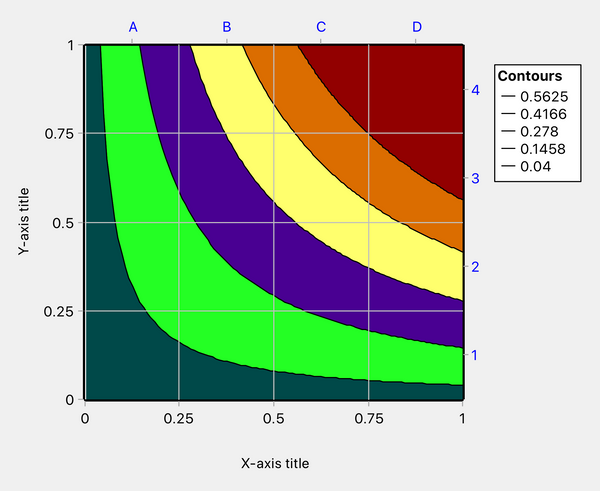Figure 1: Risk graph

We convert the risk graph to a risk matrix using the Predominant Color algorithm (one of several algorithms in Quick Risk Matrix). This colors each cell split by iso-risk contours according to the color that predominates in the cell.Figure 2: Risk matrix

The above risk matrix has been contrived to be identical to one developed by Li et al. using their Sequential Updating Approach (SUA) with an alpha value of 0.8.

The performance benchmarks for the above matrix were calculated in Quick Risk Matrix based on 100,000 points generated assuming probability and consequence to be uniformly distributed and independent (as per Li et al.):

• Points mapped accurately to risk priority level 65%
• Points with overestimated risk priority level 15%
• Points with underestimated risk priority level 20%
• PEE 11%
• PRR 1.6%

The mapping accuracy is poor but the ability to rank pairs of risks as indicated by PEE and PRR is quite good. Cox's claim that typical risk matrices have an error rate in ranking pairs of risks in excess of 90% is not borne out.

Cox stated in his paper that "For risks with negatively correlated frequencies and severities, they [risk matrices] can be 'worse than useless,' leading to worse-than-random decisions." To test this claim, we will run our performance simulation again but this time assuming that probability and consequence are negatively correlated with Spearman correlation coefficient of -0.8. A small subset of the generated points is shown below overlaid on the risk matrix:Figure 3: Risk matrix overlaid with a sample of negatively correlated points

The performance benchmarks for the matrix with the negatively correlated risks are

• Points mapped accurately to risk priority level 64%
• Points with overestimated risk priority level 16%
• Points with underestimated risk priority level 20%
• PEE 20.2%
• PRR 2.3%

Our results are almost the same as before for mapping accuracy but somewhat worse for PEE and PRR. However, Cox's claim that the risk matrix should lead to "worse than random decisions" with negatively correlated risks is clearly disproved.

We shall now investigate the effect of using fewer colors. Eliminating the smallest iso-risk contour produces the risk matrix shown below. This is identical to the risk matrix produced by Li et al. using SUA with an alpha value of 0.83.Figure 4: Risk matrix with the number of colors reduced to five

The performance benchmarks for the matrix with the number of colors reduced to five (risks treated as independent as per Li et al) are shown below with the values from the inital analysis in parentheses.

• Points mapped accurately to risk priority level 77% (65%)
• Points with overestimated risk priority level 4% (15%)
• Points with underestimated risk priority level 19% (20%)
• PEE 11% (11%)
• PRR 1.1% (1.6%)

Eliminating one color has substantially increased the mapping accuracy, raising it from 65% to 77%, with the PEE the same as before and the PRR slightly better.

At this point, we shall stop emulating the designs obtained with SUA by Li et al. This is because, as the SUA alpha value increases, the lowest risk priority level occupies more and more of the chart area. For example, for an alpha value of 0.95, the SUA-based design given by Li et al. results in every cell being in the lowest risk priority level except for the top right cell. Such a matrix would not be at all useful in practice. Since the alpha value dictates not only the number of colors but also the risk matrix coloring pattern, it appears that the method of Li et al. cannot take into account specific iso-risk contours values for the purpose of defining thresholds between risk priority levels. Instead, the coloring pattern implies the iso-risk contour values! This makes SUA unsuitable as a basis for risk matrix design because it does not allow the designer to specify key parameters. Cox's design approach has a similar deficiency. His axioms are so restrictive as to give little choice over the coloring pattern and therefore cannot reflect the designer's choice of risk thresholds.

So now we reduce the number of colors to three by eliminating two more iso-risk contours. We chose the contours to retain so that the domain was divided into three very roughly equal areas.Figure 5: Risk matrix with the number of colors reduced to three.

With the number of colors reduced to three, the performance benchmarks are as follows (with the values from the 6-color design in parentheses):

• Points mapped accurately to risk priority level 88% (65%)
• Points with overestimated risk priority level 1% (15%)
• Points with underestimated risk priority level 11% (20%)
• PEE 11% (11%)
• PRR 0.5% (1.6%)

The improvement in mapping accuracy due to reducing the number of colors is once again substantial. PRR has also improved. PEE is about the same.

### Summary of Numerical Results

We performed a few more calculations in addition to those described above and summarize the results below.

Spearman correlation coefficient = 0

 Benchmark 6 colors 5 colors 3 colors Mapping accuracy 65% 77% 88% PEE 11% 11% 11% PRR 1.6% 1.1% 0.5%

Spearman correlation coefficient = -0.8

 Benchmark 6 colors 5 colors 3 colors Mapping accuracy 64% 74% 84% PEE 20% 20% 20% PRR 2.3% 2.3% 1.0%

## Conclusions

Quick Risk Matrix (Premium) includes a risk matrix performance benchmarking tool to calculate several statistics:

• Mapping accuracy
• Probability of Ranking Reversal (PRR)
• Probability of Elimination Error (PEE)

The main purpose of a risk matrix is to map probability and consequence categories to risk priority levels. The mapping accuracy benchmark is an indicator of how well a matrix can do this. It is useful for comparing alternative risk matrix designs.

The Probability of Rank Reversal (PRR) is the probability that a pair of risks, with the two risk points located in different risk priority levels, is ranked incorrectly by the risk matrix. For our worked examples, the PRR was small (0.5% to 2.3%). For well-designed risk matrices, it is our experience that PRR is typically small.

The Probability of Elimination Error (PEE) is the probability that a pair of risks located anywhere in the risk matrix will be ranked incorrectly. Now, the risk matrix on its own is incapable of ranking a pair of risks when both points lie in the same risk priority level. When the two risks have equal risk priority level, the decisionmaker must break the tie by means of supplementary calculations. When calculating PEE, Quick Risk Matrix assumes that the decisionmaker would use the Geometric Center Method (explained above) for breaking ties. Thus, PEE is an indicator of how well a decisionmaker might do in ranking pairs of risks with the aid of the risk matrix and some supplementary calculations.

We consider PEE to be a statistic of lesser importance since, in the real world, risks are not chosen for elimination or reduction solely on the basis of magnitude. The cost of elimination or reduction plays an important role. If there are many small risks that can be inexpensively treated, the cumulative risk reduction may be greater than what could be achieved by treating one or two larger but more intractable risks.

In our worked examples, we found a reduction in risk matrix performance when probability and consequence are negatively correlated. The reductions were modest and not sufficient to detract from the usefulness of the risk matrix.

Cells split by iso-risk contours have ambiguous risk priority level and create mapping errors. Our worked examples illustrate that the mapping accuracy benchmark typically improves as the number of colors in the matrix reduces. This is to be expected since fewer cells will be split by iso-risk contours when the number of colors is reduced. We recommend that the number of colors is not more than needed by the organization's risk management philosophy. For many organizations, three colors may be sufficient, representing risks too high to be tolerated, risks so low that they are broadly acceptable, and risks that are acceptable provided that they have been reduced to as low as is reasonably practicable (ALARP).

# References

Ref. 1. Cox, L.A., What’s Wrong with Risk Matrices? Risk Analysis, Vol. 28, No. 2, 2008.

Ref. 2. Xing Hong, Risk Matrix Analysis Using Copulas. Dissertation, The George Washington University.

Ref. 3. Li J., Bao C., Wu, D. How to Design Rating Schemes of Risk Matrices: A Sequential Updating Approach. Risk Analysis, Vol. 38, No. 1, 2018.

]]>
https://riskmatrix.co/blogs/risk-matrix-knowledge-bank/nasa-risk-matrix-an-example-of-how-not-to-design-a-risk-matrix 2018-03-27T20:55:00-05:00 2018-05-26T20:35:33-05:00 How Not to Design a Risk Matrix - NASA's Risk Matrix Peter Carr More

]]>
NASA's activities include building spacecraft, instruments and new technology to study Earth, the Sun, the solar system and the universe.

## NASA's Standard Risk Matrix

In assessing the risks to its projects, NASA uses a standard risk matrix as shown in Figure 1 (reference NASA Goddard Procedural Requirements GPR 7120.4D, 2012).

The likelihood and consequence categories are ordinal with labels 1, 2, ... 5 along each axis. Ordinal means that the category labels do not indicate the true magnitude of the variables but only the direction of increase. We shall revise the category labels to A, B, C, ... for the horizontal axis because a reference to a cell by its column and row labels will be clearer when written as, for example, D2 rather than as 42 (D2 reminds us which digit represents the column and which represents the row). With this revision to the labels, NASA's standard matrix is as shown in Figure 1.Figure 1: NASA standard risk matrix (redrawn by us)

In case you are wondering why Figure 1 uses those particular shades of red, yellow and green to denote the risk priority levels, rather than the corresponding primary colors, it is to provide better differentiation for people with one of the three common forms of color blindness.

NASA applies the risk matrix shown in Figure 1 to different types of consequence, such as safety, technical, schedule, cost, etc. NASA associates the five consequence categories with detailed descriptions but rarely defines the consequence categories quantitatively. As we explain elsewhere in this blog, it is not possible to perform meaningful calculations with ordinal scales and, if we want to design a defensible risk matrix, we need to use ratio scales.

Fortunately for our audit of its risk matrix, NASA has provided ratio scales (as well as ordinal scales) for two types of consequence – budget increase and operational cost threat –  and we are going to use these scales to carry out logical consistency checks on their risk matrix. In making these checks, we shall assume that NASA calculates risk as the product of probability and consequence, which is by far the most common method and nothing in NASA's documentation appears to suggest anything different.

The NASA consequence categories for budget increase are <2%, 2–5%, 5–10%, 10–15%, and >15%. For operational cost threat, they are <\$1M, \$1M–\$5M, \$5M–\$10M, \$10M–\$60M, and >\$60M. Notice that the first and last categories are open at one end. We shall make some reasonable assumptions for the values of the missing endpoints since we shall want to draw the matrices to scale.

NASA likelihood categories are 2%–10%, 10%–25%, 25%–50%, 50%–75%, and >75%. We will close the last category by replacing it with 75%–100%.

## NASA's Risk Matrix Applied to Budget Increase

Figure 2 shows NASA's risk matrix when applied to budget increase and when drawn to scale. Note that we have used a logarithmic scale for the horizontal axis and a linear scale for the vertical axis. The choice of linear or logarithmic scale is made according to which is better at preventing overlapping of labels and has no effect on risk matrix design.Figure 2: NASA risk matrix for budget increase when drawn to scale

We detected an inconsistency in the risk matrix for budget increase. Look closely at cells B3 and D2. As shown in Figure 3 below, the quantitative risk in both these cells ranges from 0.5 at the bottom left corner of the cell to 2.5 at the top right corner. Yet B3 has a lower risk priority level than D2. Both cells should be of the same color since they contain an identical range of risks.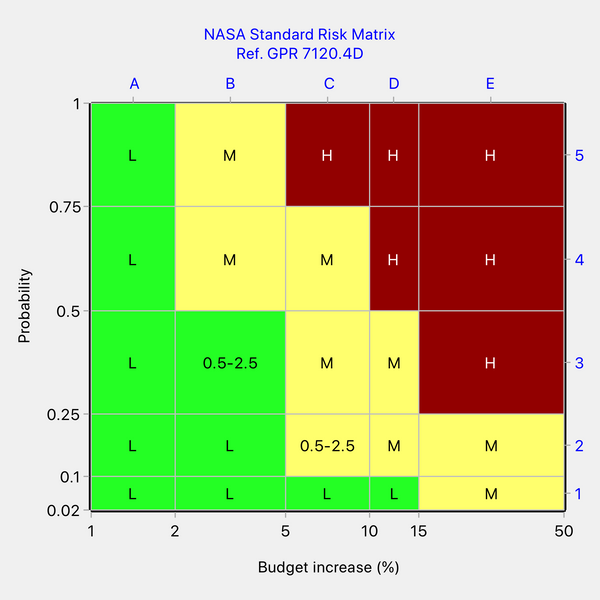Figure 3: Showing an inconsistency in NASA's risk matrix for budget increase

We conclude from Figure 3 that the coloring of the matrix is inconsistent with its underlying ratio scales in the case of the consequence type "budget increase".

## NASA's Risk Matrix Applied to Operational Cost Threat

Now let's look at the NASA standard risk matrix when applied to operational cost threat. We can find inconsistencies in this risk matrix too, but it is not as straightforward to do so as in the previous case. The matrix can be assumed to be underlain by two undisclosed iso-risk contours that define the boundaries between risk priority levels. To identify inconsistencies, we need to know NASA's strategy for determining the color of a cell split by a contour. A split cell contains some risk points that lie in the risk priority level below the contour and other points that lie in the risk priority level above the contour. We shall assume that NASA's strategy is to apply the color above the contour to the split cell. Quick Risk Matrix provides a choice of algorithms for coloring split cells, one of which is called the "round-up" algorithm. In effect, we are assuming that NASA is using the round-up algorithm. With this algorithm, the color of a split cell is determined only by the risk value at the top right corner of the cell, which is the maximum possible value for a risk point in that cell. With this assumption, we can now proceed to look for inconsistencies considering only the maximum risk value in each cell.

Figure 4 below shows NASA's matrix for operational cost threat with the maximum risk value written into several of the cells.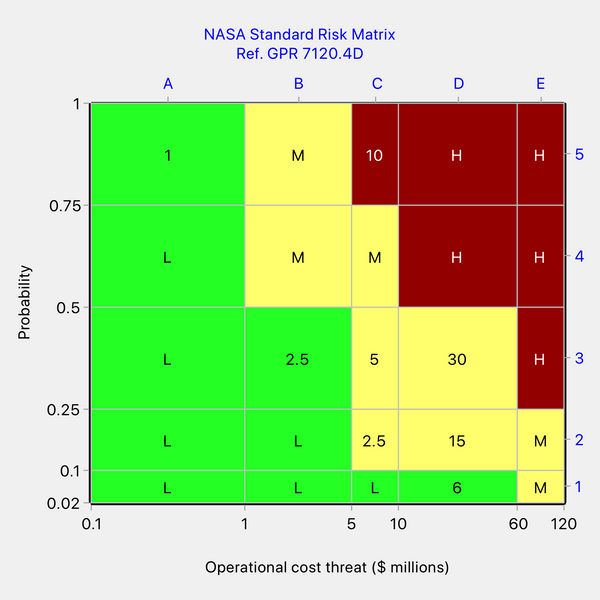Figure 4: NASA's risk matrix for operation cost threat with the maximum risk value written into several cells

Examining Figure 4, cell C2 (Medium risk) has a maximum value of 2.5, which is identical to the maximum value for cell B3 (Low risk) and less than the maximum value for cell D1 (Low risk). We can see that NASA is not weighting for higher consequences because D1 (Low risk) is higher consequence than C2 (Medium risk). We could resolve this inconsistency in various ways. One way would be to assume that C2 is correctly colored. Then the color of cells B3 and D1 should be the same as the color of cell C2, ie. cells B3 and D1 should be uprated from Low risk to Medium risk.

Still looking at Figure 4, cell C5 (High risk) has a maximum value of 10, which is less than the maxima for cells D3 and D2 (maxima 30 and 15, respectively, and Medium risks). Once again, we can see that NASA is not weighting for high consequence since C5 (High risk) has lower consequence than D2 or D2 (Medium risk). Again, the inconsistency could be resolved in various ways. If we assume cells D3 and D2 (Medium risk) are correctly colored, then cell C5 would need to be downrated from High to Medium risk.

## The Corrected Risk Matrices

It is emphasized that there many ways to revise the two risk matrices to make them internally consistent. We gave examples of possible corrections in the previous sections. Applying those corrections, the revised risk matrices are as shown in Figures 5 and 6 below.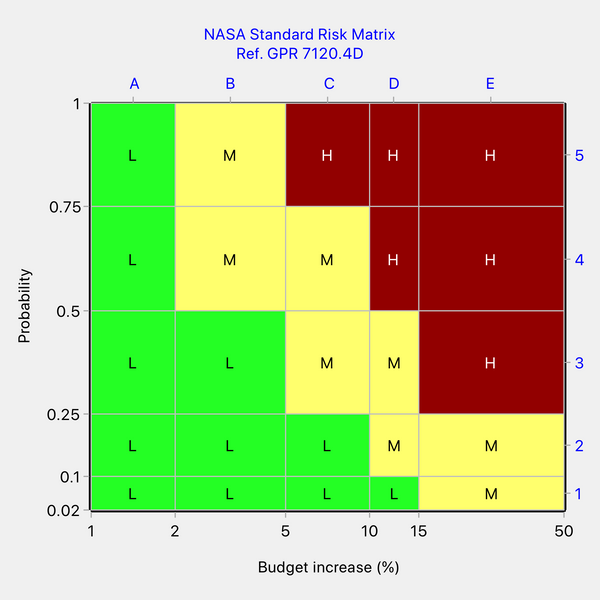Figure 5: One possible revision to NASA's risk matrix for budget increase to make it self-consistent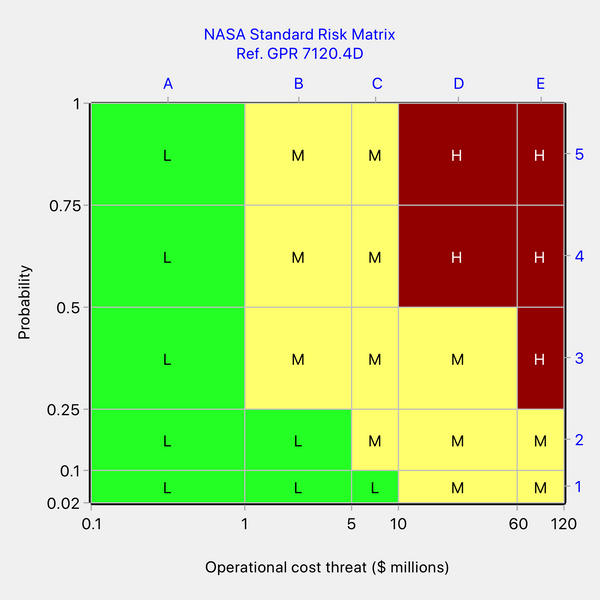Figure 6: One possible revision to NASA's risk matrix for operational cost threat to make it self-consistent

A matrix identical to that in Figure 5 may be obtained in Quick Risk Matrix by using the round-up algorithm and iso-risk contour values of 1.25% and 5%.

A matrix identical to that in Figure 6 may be obtained in Quick Risk Matrix by using the round-up algorithm and iso-risk contour values of \$1.25M and \$30M.

Although we made both matrices self-consistent, we had to adopt different coloring patterns to do so. This illustrates that changing the axis scales of a risk matrix will, in general, also change the required coloring pattern.

## Discussion and Conclusions

We can learn many lessons from this review:

• For the two consequence types for which we were able to audit NASA's risk matrix because ratio scales had been defined, we demonstrated that the risk matrix coloring is inconsistent with the underlying ratio scales (based on the usual definition of quantitative risk as the product of probability and consequence). The anomalies cannot be explained by assuming that NASA is weighting for higher consequences.
• There may be additional design imperfections in the two matrices but it is difficult to say with certainty without knowing the design intent, in particular, the intended values of the two risk thresholds separating the three risk levels.
• When only ordinal scales are given, the associated matrix will be largely unverifiable and therefore cannot be regarded as technically defensible. This applies to most of the scales employed by NASA. Sometimes ordinal scales look like numbers (e.g. 1...5), but they are really just labels and calculations should never be performed with them.
• The same risk matrix coloring cannot be blindly assumed to apply when the underlying scales are changed. If the scales (or the risk thresholds) are changed, the appropriate coloring pattern will change in general.
• If it is desired to use the same coloring pattern with different scales and risk thresholds, this will only be possible when the axis scales and risk thresholds are changed proportionately. Arbitrary changes to the scales or thresholds will invalidate the coloring.
• Even organizations with impressive technology such as NASA are not immune from making errors when designing risk matrices.
• Errors of design can be avoided by using Quick Risk Matrix, which guarantees a self-consistent risk matrix every time.
]]>
https://riskmatrix.co/blogs/risk-matrix-knowledge-bank/how-not-to-design-a-risk-matrix-1 2018-03-27T19:02:00-05:00 2018-05-26T19:25:09-05:00 How Not to Design a Risk Matrix - A Public Health Risk Matrix Peter Carr More

]]>
In a another post, we discussed a concern expressed by Cox (Ref. 1) that risk matrices may be "worse than useless" when probabilities and consequences are negatively correlated. We showed in that post that the concern only arises in highly contrived situations.

A paper by Vatanpour et al. of the University of Alberta (Ref. 2) is particularly interesting because it attempts to investigate Cox's concern by reference to real-world data from the public health field. The authors conclude that Cox's concern is valid, but we shall show in this post that their conclusion is illusory and due to use of a poorly designed risk matrix.

The risk matrix used by Vatanpour et al., redrawn with axes to scale, and displaying ratio scales as well as categories, is as follows: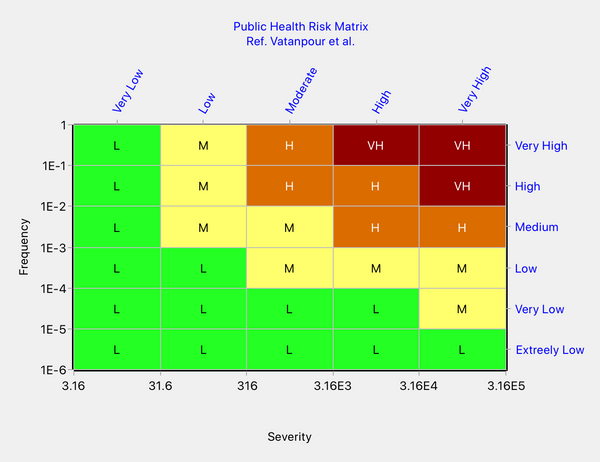Figure 1: Risk matrix of Vatanpour et al.

The frequency scale is as stated by Vatanpour et al. The severity scale was not explicitly given but we noted that severity was scored as 10, 100, 1000, 1E4 and 1E5 for the five categories, respectively. Our tick marks were calculated to place the given severity scores approximately at the geometric mean of each severity category. For example, the geometric mean of 3.16 and 31.6 is approximately 10. The matrix classifies risks into four risk priority levels, ranging from Low to Very High.

Vatanpour et al. plotted the risks associated with a number of blood-borne infectious diseases on their matrix. The plotted points exhibited negative correlation, as would be expected (since more severe consequences tend to be less frequent). The Spearman correlation coefficient was -0.81. Due to a paucity of real-life data, they also included four risk points that were fictitious but chosen to fit with the general trend. The artificial data points were called Datum 1 through Datum 4.

The authors observed that Datum 2 (empirical risk = 0.21) and Datum 4 (empirical risk = 0.5) were rated as Medium risk whereas TT Virus (empirical risk = 10) was rated as Low risk - see Figure 2 below. This type of anomaly, where larger risks are ranked lower than smaller risks, is called rank reversal.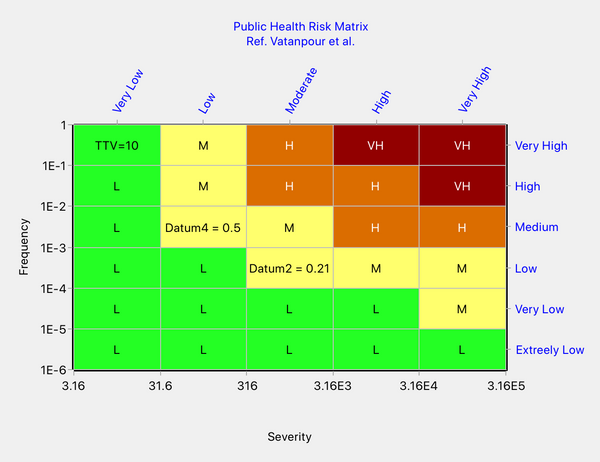Figure 2: The rank reversal anomaly observed by Vatanpour et al.

Vatanpour et al. attempt to explain the anomaly as follows:

"The generated data points 2 and 4 have estimated risk values of 0.21 and 0.50 and both are categorized in Figure 5 as medium risks. When compared with TT virus, which was categorized as a low risk in Figure 5, we find that it has an estimated (according to Equation (4)) risk of 10. This anomaly illustrates the concern posed by Cox, that the risk assessment matrix provides a risk categorization (color code) that is incorrect in relation to an empirical calculation of the risk . Although we had to resort to generating data from an empirical relationship derived from experiential frequency estimates, we have found that the theoretical concern of Cox can be demonstrated for hazard data derived from authentic experience."

It is surprising that the authors did not discuss the possible alternative explanation that the rank reversal error might be due to inadequate risk matrix design. It is also surprising that this possibility was not picked up in the journal's peer review process.

Consider the cells in which Datum 2 and Datum 4 are located. Points in either of these cells can have risks ranging from 0.0316 at the bottom left to 3.16 at the top right. Compare with the cell containing the data point relating to TT virus (TTV). The risk range for the cell containing the TTV data point is 0.316 to 31.6, i.e. risks in this cell are 10 to 100 times higher than in the cells containing Datum 2 and Datum 4. Therefore, if the yellow coloring is correct for the latter cells, then the cell containing the TTV data point cannot be green but must be at least yellow.

Thus, the authors have not validated the theoretical concern of Cox but instead have vividly demonstrated a common error in risk matrix construction, which is to fail to properly account for the axis scales when determining the matrix coloring pattern.

We will redesign the authors' risk matrix using Quick Risk Matrix. Unfortunately, the authors did not state the quantitative values defining the four risk zones. We will assume that the three iso-risk contours separating the four risk zones take the values 3160, 31.6 and 0.316. Thus, each risk threshold is a factor of 100 lower than the one above it. This gives a risk graph as shown below. Quick Risk Matrix is based on the concept that one develops a risk graph and then converts it to a discrete risk matrix. Several different algorithms are provided for making the conversion.Figure 3: Risk graph to serve as the basis for the redesign of the risk matrix

Since this risk matrix is for use in connection with the important safety issue of bloodborne disease, it is prudent to use the Round-Up algorithm, which colors each split cell according to the highest risk zone in it. The Round-Up algorithm ensures that any errors will be the safe side, i.e. the risk level will never be underestimated. With the Round-Up algorithm, we arrive at the following design: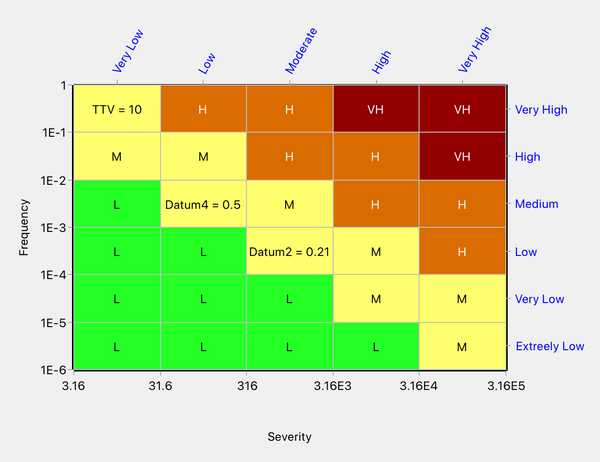Figure 5: Redesign in Quick Risk Matrix based on the Round-Up algorithm

Note that our redesign has eliminated the rank reversal anomaly observed by Vatanpour et al. All three data points are now ranked the same, i.e. medium risk.

Our redesign has a coloring pattern substantially different to that of Vatanpour et al. This suggests that if we look hard enough at the design of Vatanpour et al., we should be able to detect some inconsistencies. This is indeed the case. For example, compare the cells in column 2, row 6, and column 3, row 5. Points in both these cells lie in the risk range 3.16 to 316. In the design of Vatanpour et al. (see Figure 1 or 2), these two cells have different colors. Since these cells have identical risk range, they should have the same color. In fact, with these axes, where the tick mark values increase by a factor of 10 as we move across the columns or rows, the risk range is identical for all cells lying on any diagonal line having a slope of -1. The color should, therefore, be the same for all cells lying on such a diagonal. Informed by this observation, we can see that the risk matrix design of Vatanpour et al. contains multiple incorrectly colored cells.

We can benchmark the accuracy of our redesign using the performance simulation tool in Quick Risk Matrix Premium. We assume that the frequency and severity have a log-uniform distribution with a Spearman correlation coefficient of -0.81 (the value given by Vatanpour et al.). We find that the Round-Up algorithm is predicted to achieve a mapping accuracy of 76% with all errors on the safe side. If we simulate for pairs of random points, the percentage error in ranking the two points of a pair is found to be 0.7% if the two points lie in different colored regions and 23% if they do not necessarily lie in different colored regions. (We explain in another post how all these benchmarks are calculated.)

Note that the benchmarks do not necessarily accurately predict real-world performance but are intended only for comparing different risk matrix designs.

Our final illustration shows a sample of points generated, with the above-described negative correlation, plotted on the risk matrix produced by the Round-Up algorithm. A substantial proportion of the generated points lie in cells split by iso-risk contours, which explains why the mapping accuracy is relatively low at 76%. Mapping errors are inevitable when using a risk matrix rather than a risk graph but, if we are concerned about them, we can use the round-up algorithm to ensure that errors are on the safe side.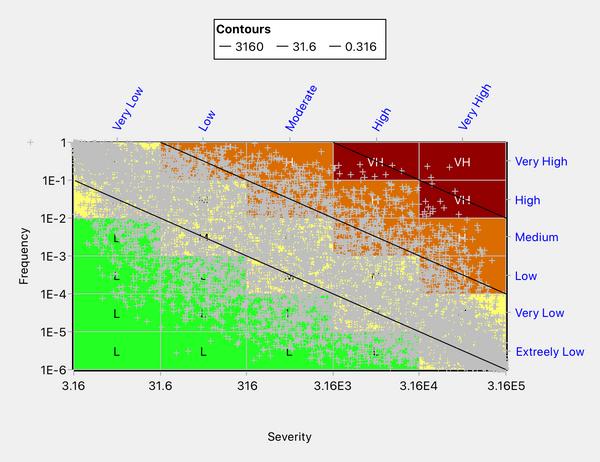Figure 6: A sample of negatively correlated points used to benchmark the accuracy of the risk matrix

It is concluded that the risk matrix of Vatanpour et al. did not validate Cox's concern relating to negative correlations but instead demonstrated a common error in risk matrix design, namely not adequately considering the axis scales.

Ref. 1, Cox, L.A., What’s Wrong with Risk Matrices? Risk Analysis, Vol. 28, No. 2, 2008.

Ref. 2. Vatanpour S., Hrudey S.E., and Dinu, I., Can Public Health Risk Assessment Using Risk Matrices Be Misleading? Int. J. Environ. Res. Public Health 2015, 12, 9575-9588.

]]>
https://riskmatrix.co/blogs/risk-matrix-knowledge-bank/how-not-to-design-a-risk-matrix-national-health-service-risk-matrix 2018-03-27T17:58:00-05:00 2018-05-26T19:24:08-05:00 How Not to Design a Risk Matrix - National Health Service (UK) Risk Matrix Peter Carr There are many ways in which to design a poor risk matrix, but one method is used so often that it deserves its own article. This unsound method involves the use of ordinal scales for likelihood and consequence and a pretense that the ordinal scales (which are essentially just labels) can express the magnitude of the likelihood and consequence variables.

More

]]>

There are many ways in which to design a poor risk matrix, but one method is used so often that it deserves its own article. This unsound method involves the use of ordinal scales for likelihood and consequence and a pretense that the ordinal scales (which are essentially just labels) can express the magnitude of the likelihood and consequence variables.

A case in point is the risk matrix of the UK National Health Service (NHS). (Reference. A Risk Matrix for Risk Managers, NHS, National Patient Safety Agency, 2008. ) Introduced in 2008, the risk matrix continues to be used by health organizations across the United Kingdom: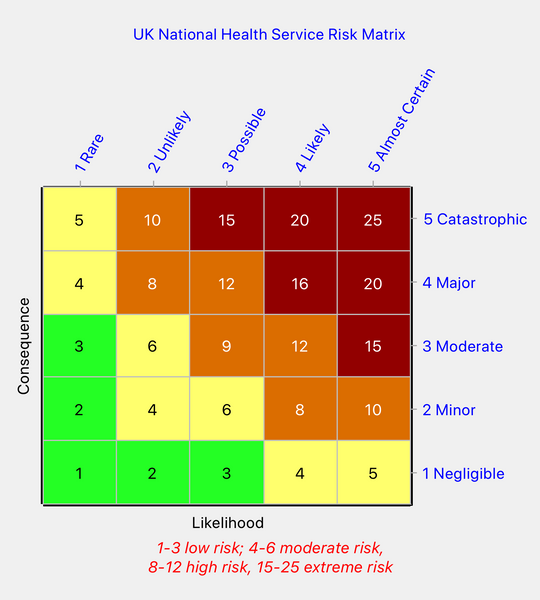Figure 1: NHS risk matrix

Figure 1 shows the ordinal scales, which range from 1 to 5 on each axis. It also shows the score assigned by the NHS to each cell, which is the product of its column and row ordinal values, and the risk scores that define the four risk priority levels ("low," "moderate," "high" and "extreme" risk).

The NHS uses the same matrix for 10 types of consequence. It terms a consequence type a "domain." Two examples of domains are "Impact on the safety of patients, staff or public ..." and "Adverse publicity/ reputation." It also uses the same matrix in relation to three different likelihood scales: (1) broad linguistic descriptors of frequency (no numbers), (2) time-based descriptors ("daily", "weekly", "monthly", etc.), and (3) probability descriptors ("<0.1%", "0.1 to 1%", "1 to 10%", etc.).

It is not explained why the risk matrix coloring should be expected to be the same for 10 different consequence domains and three different likelihood scales. And nor is it explained why the thresholds between different risk priority levels should also be the same regardless of the consequence domain or frequency scale.

Rational risk matrix design depends on the use of ratio (quantitative) scales for likelihood and consequence. If we are to audit the NHS matrix, we need to identify the ratio scales that underlie it. Unfortunately, for most of the consequence domains, the descriptors are linguistic, not quantitative. There are a few exceptions, however, so all is not lost.

We will consider the consequence domain called "business objectives/projects." It appears that this domain is intended to be used in conjunction with the probability descriptors of likelihood. Although we can extract most of the axis tick mark values from the NHS documentation, the upper and lower bounds of the scales are undefined, so we are forced to make some reasonable assumptions. For example, the "Catastrophic" consequence is defined as a budget overrun greater than 25%, so we have assumed that that category could range from 25% to 100%. It is not widely appreciated that the bounds do matter and can affect the coloring of the top and bottom rows and the left and right columns. So, with reasonable assumptions, the scales for this case look something like this (plotted on log scales):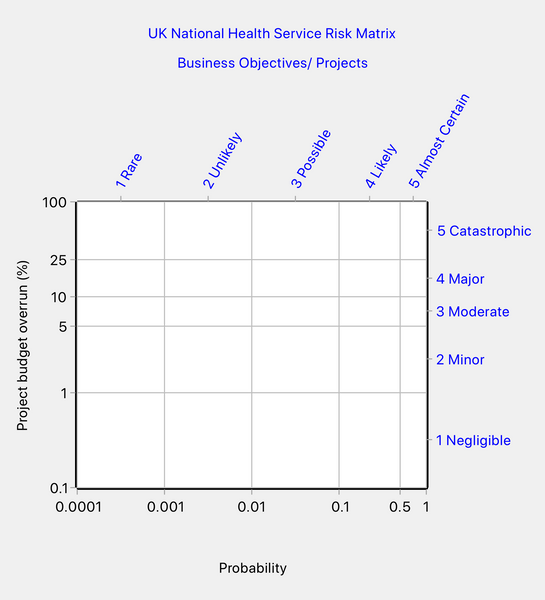Figure 2: NHS risk matrix - scales for business objectives/projects

To design a risk matrix, we also need to know the values of the iso-risk contours that separate the different risk priority levels. That information was not provided but we can make some assumptions and sensitivity studies. We quickly discover that any design we produce using the above scales is not going to be symmetrical, in contrast to the NHS matrix which is completely symmetrical.

We assume in this audit that risk should be calculated as the product of probability and consequence, as is conventional. We can be confident this is how the NHS views risk since we have seen that the NHS multiplies row and column numbers to obtain a surrogate for risk and so is definitely not using a more sophisticated definition.

One attempt to reproduce the NHS matrix looks as shown below in Figure 3. We used the Predominant Color algorithm of Quick Risk Matrix for this design. With that algorithm, each cell split by an iso-risk contour takes the color of the risk priority level that occupies the largest area of the cell.Figure 3: An attempt to reproduce the NHS risk matrix

We arrived at the matrix in Figure 3 by positioning the two lowest contours so that columns 1 and 2 match the NHS design and by positioning the highest contour so that row 5 matches the NHS design. But it is evident that we cannot match the entire matrix and no amount of playing with the contour values is going to produce a complete match. We also tried other coloring algorithms with no better result.

Thus, it appears that the standard NHS matrix is not consistent with this particular set of axis scales. That suggests that we might be able to find inconsistencies in the NHS matrix and indeed we can. Look at the two cells marked up in the following figure: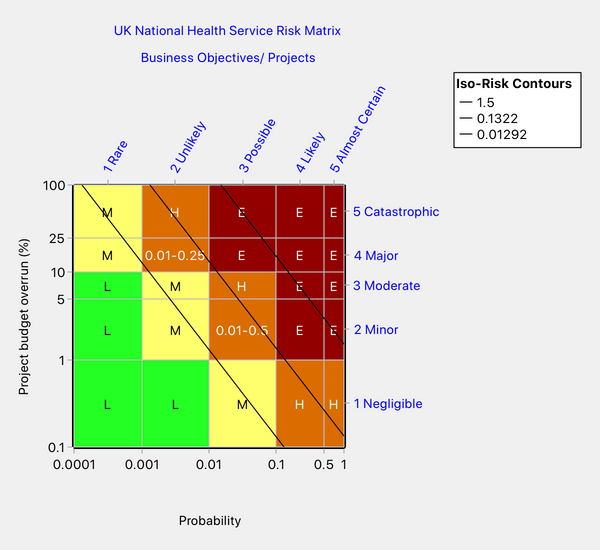Figure 4: Two cells in our redesign that the NHS risk matrix (Figure 1) rates inconsistently

Our design in Figure 4 identifies two cells in risk priority level "High" that the NHS matrix in Figure 1 colors inconsistently. Note that he cell in column 2, row 4, contains points corresponding to risks ranging from 0.01 to 0.25, while the cell in column 3, row 2, contains risks from 0.01 to 0.5. The NHS risk matrix (Figure 1) ranks the former cell as "High" risk and the latter as "Moderate" risk. Since the two cells have the same lower bound of 0.01 but the latter has a greater upper bound, then the latter cell cannot have a lower risk rating than the former, as it does in the NHS matrix.

There are other inconsistencies in the NHS matrix but we shall be content to just give this one example.

Now suppose we accept Figure 4 as the "correct" coloring (we put "correct" in quotes because there is more than one possible design). Figure 4 is internally consistent, unlike the NHS matrix. Now suppose we consider a different consequence domain, with an axis having different subdivisions and/or with different values for the iso-risk contours separating the risk priority levels. There is a very good chance that we will find that a different coloring pattern would be required.

One may change an axis by a scaling factor without changing the coloring pattern, provided the risk thresholds are scaled by the same factor. For example, it makes no difference to the risk matrix design whether we use dollars or pesetas for a money axis. The same is true for scaling factors generally. But once the relative widths of the axis subdivisions differ, then the required coloring pattern will likely differ. The safest approach is to use Quick Risk Matrix to verify the matrix design in relation to all the scales with which it is to be used.

]]>
https://riskmatrix.co/blogs/risk-matrix-knowledge-bank/how-to-design-a-risk-matrix 2018-03-27T17:35:00-05:00 2018-06-06T17:08:05-05:00 How to Design a Risk Assessment Matrix (Correctly) Peter Carr More

]]>
This article outlines the methodology used in Quick Risk Matrix for the development of risk matrices, also known as risk assessment matrices.

A risk matrix is a chart in which one axis is subdivided to indicate categories of likelihood while the other axis is subdivided to indicate categories of consequence. The cells of the matrix are colored to indicate how any given pair set {likelihood-category, consequence-category} maps to a risk category. We term the risk categories "risk priority levels."

Likelihood may be expressed as probability or as frequency.  We will use the term probability from this point on, but it should be remembered that it could equally well be frequency.

Risk matrices are used in risk assessments. Potential unwanted events are identified. Each event is assigned to a probability category and to a consequence category. The probability category indicates the likelihood that the event will occur. The probability category indicates the severity of the consequences. For a given pair of categories, the risk matrix indicates the risk priority level. The organization's risk management procedures typically make use of the risk priority levels when specifying acceptability, urgency, priority, required level of management attention, etc.

The most popular size for a risk matrix is 5 x 5 but some organizations are using larger risk matrices with up to 10 rows and columns. Quick Risk Matrix imposes no limit on the number of rows or columns.

An example risk matrix is shown below. The categories are labeled and also defined by their numeric ranges. Note that we often use E-notation to keep the chart tick labels compact so that they do not overlap.

When the probability or consequence variable spans a large range, the axis will use a logarithmic scale for legibility. This example uses log scales. The choice of logarithmic or linear scale makes no difference to the coloring pattern of the risk matrix; it is only a legibility issue.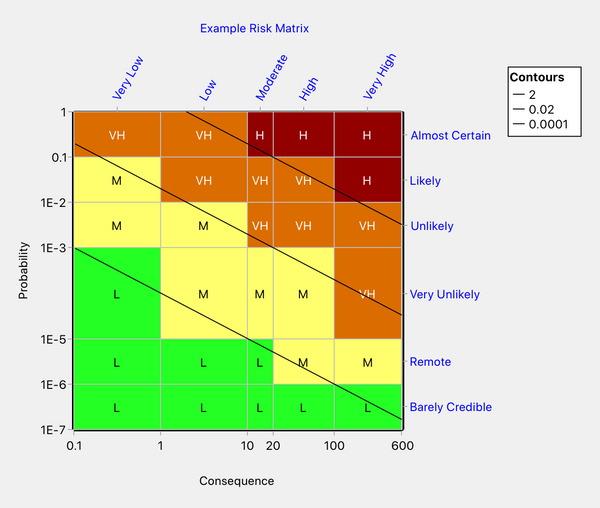Figure 1: Example risk matrix

In the figure above, the probability and consequence categories have been defined using numeric scales (e.g. 0.1, 1, 10, …) and also with labels (e.g. Very Low, Low, ...).

The numeric scales are known as "ratio" scales, because we can compare the sizes of quantities expressed on these scales (i.e. calculate ratios). Most scientific and engineering measurements are made on ratio scales.

The category labels are known as “ordinal” scales. An ordinal scale shows the order in which a variable increases but often tells us nothing about its magnitude.

Some organizations omit ratio scales from their risk matrices and use only ordinal scales. A risk matrix that uses only ordinal scales is almost unverifiable. The only consistency check that can be made is whether a cell above and/or to the right of another cell has an equal or higher risk priority level. So, for example, referring to the above figure, the cell {High, Likely} must have a risk priority level equal to or higher than the cell {High, Unlikely}, because both the consequence and the probability in the former cell are equal to or higher than in the latter cell. But, there is no way to verify the ranking of a pair of cells when one cell is to the right and below another cell. For example, the cell pair {High, Likely} and {Very High, Unlikely} cannot be ranked because the former has lower consequence but higher probability than the latter, and there is no way of knowing whether the consequence or the probability dominates.

A further disadvantage of purely ordinal scales is that linguistic terms like “low”, “moderate”, “likely” and so on will be interpreted very differently by different people.

Since a risk matrix that uses only ordinal scales cannot be fully verified, it follows that its use cannot normally be defended. There is an exception to this rule, which we will describe below.

The exception is that a matrix with a ratio scale for probability and an ordinal scale for consequences is sometimes reasonable when consequences are difficult or controversial to measure. A prime example might be a risk matrix for workforce safety. Let’s say the consequence scale has ordinal categories A–E, the workforce population size is 200, and the categories are defined as “A. One or more injuries, not severe,” “B. One or more severe, possibly permanently disabling, injuries,” “C. 1–4 fatalities,” “D. 5–50 fatalities,” “E. 51–200 fatalities.” The difficulty here is that the measurement units are mixed, being number of injuries at the lower end and number of fatalities at the higher end. One way round this difficulty is to temporarily assign a ratio scale, either based on the monetary value of averting an injury/fatality or based on treating an injury as a fraction of a fatality. Let’s say we treat an injury as a fraction of a fatality. Then the tick marks on the ratio scale might be (for example) 0, 0.5, 1, 5, 50, 200. (If using a log scale, zero should be replaced with a small non-zero number since zero cannot be plotted on a log scale.) The matrix is then designed based on the temporary consequence scale and a suitable ratio scale for probability. Finally, the temporary consequence scale is hidden since the injury to fatality equivalence is uncertain and possibly controversial.

When ratio scales are used, the risk matrix may be developed with mathematical precision. Quick Risk Matrix requires the use of ratio scales, even if only temporary as described in the previous paragraph.

When setting up a ratio scale for consequences, any units of measurement relevant to the type of risk you are assessing may be used, for example, dollars, number of fatalities, volume of chemical discharged to the environment, hectares of land contaminated by a spill, percentage budget overrun, weeks of schedule delay, and so on.

Another requirement for objective risk matrix design is clarity on how risk is to be measured. There is a high degree of consensus that risk is to be computed by combining consequence and likelihood (see for example ISO Guide 73:2009). By far the most common method of forming the combination is to multiply the values of the probability and consequence variables (i.e. risk = probability times consequence). Note that the product of the two variables is the statistical "expected value," i.e. the average value of the consequence measure in a large number of identical situations. We call "expected value" "expected loss" when the consequences are detrimental. In a future article, we will look at an alternative to expected value that is available in the Premium edition of Quick Risk Matrix.

A "risk graph" (our term) is a logical predecessor to a risk matrix. The risk graph uses the same axes as the risk matrix. On it, we plot contours of equal risk (iso-risk contours) to define the boundaries between risk priority levels. The risk graph corresponding to our example risk matrix is presented below. Note that because we have used log-log scales, the risk contours are straight lines.Figure 2: Risk graph

A note on terminology. What we term a risk graph should not be confused with the quite different type of chart with the same name in the IEC 61511-3 standard on functional safety of safety instrumented systems. We thought about calling the above type of graph a probability-consequence graph but that's a mouthful and, if we did that, then perhaps we should use the term probability-consequence matrix in place of risk matrix. On balance, it seemed preferable to use the term risk graph as the direct analog of the term risk matrix.

We can construct the risk matrix (Figure 1) from the risk graph (Figure 2) in two steps. We begin by coloring the cells of the risk matrix that are not split by the risk contours. These cells can be colored based on the coloring of the risk graph, which, for our example, gives the result in Figure 3 below. There may be a lot of blank cells at this stage, especially if you are trying to use many colors in a small matrix.Figure 3: Risk matrix while under construction

You can see that some of the cells of the risk matrix have a known coloring that follows directly from the risk graph. But the cells that are split by the risk contours cannot be assigned accurately to any of the qualitative risk priority levels. That is because some points in the split cells lie in one level and other points lie in another. With closely spaced risk contours, it is possible for a cell to be split not just between two but between three or more risk levels.

At this stage in the risk matrix design, when we want to color the split cells, we are forced to make an approximation. Quick Risk Matrix Premium allows this approximation to be made in any of five ways, which we briefly refer to as (1) fuzzy interface, (2) round up, (3) predominant color,  (4) round down and (5) geometric center. Quick Risk Matrix Standard offers the first three of these algorithms.

The "fuzzy interface" procedure treats the group of cells intersected by any iso-risk contour as if it is a separate risk level in its own right. If we were to do that in this example, we would end up with seven risk priority levels (colors) rather than the original four.

In the "round up" method, the color of a split cell is governed by the color of the highest risk zone in the cell. This means that the level of some risks in the cell (those risks corresponding to points lying under the highest contour intersecting the cell) will be overestimated. The merit of the rounding up method is that no risk will ever be assigned to a level that is too low. But it may be overly conservative for some circumstances, in which case one of the other methods may be used.

"Round down" colors each split cell according to the color of the lowest risk zone in the cell. It is only intended to be used when designing opportunity matrices (an opportunity matrix is similar to a risk matrix but is used to rate potential gains rather than potential losses). The round-down method is conservative for opportunity matrices.

With the "predominant color" method, the color of a split cell is taken to be the color of the risk zone that occupies the largest area within the cell. This is often the most accurate method of designing a risk matrix, but it is not conservative.

The "geometric center" method colors each split cell according to the color of the risk graph at the geometric center of the cell. This often, but not always, gives the same result as the predominant color method.

The  Premium edition of the program includes a simulation tool to enable the performance of the different coloring methods to be compared.

If we color our example matrix using the predominant color algorithm, we obtain the following result:Figure 4: Risk matrix after coloring the split cells using the predominant color algorithm

If we use the round-up algorithm, we obtain the following:Figure 5: Risk matrix after coloring split cells using the rounding-up algorithm

At this stage, the risk matrix is finished. You may hide the contours if you wish, you may hide the X- and Y-axis tick marks if they were only a construction aid, and you may export the risk graph and risk matrix to various picture formats. With the Premium edition, you may also export the risk matrix to Excel along with a risk register template.

## Summary

What makes the Quick Risk Matrix methodology so accurate is:

• The use of user-defined ratio scales for the probability and consequence axes (as opposed to ordinal scales).
• The definition of risk priority levels using contours of equal risk ("iso-risk contours"), the numeric values of which are defined by the user.
• The recognition that cells split by the iso-risk contours can only be assigned to a single risk level via an approximation — and the approximation may be made in various ways.
• The provision of several reasonable ways to make the above approximation
For more detailed information on Quick Risk Matrix, you may care to look at the program's online help file.
]]>